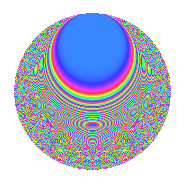# Properties

 Label 525.4.bLevel $525$ Weight $4$ Character orbit 525.b Rep. character $\chi_{525}(251,\cdot)$ Character field $\Q$ Dimension $146$ Sturm bound $320$

# Related objects

## Defining parameters

 Level: $$N$$ $$=$$ $$525 = 3 \cdot 5^{2} \cdot 7$$ Weight: $$k$$ $$=$$ $$4$$ Character orbit: $$[\chi]$$ $$=$$ 525.b (of order $$2$$ and degree $$1$$) Character conductor: $$\operatorname{cond}(\chi)$$ $$=$$ $$21$$ Character field: $$\Q$$ Sturm bound: $$320$$

## Dimensions

The following table gives the dimensions of various subspaces of $$M_{4}(525, [\chi])$$.

Total New Old
Modular forms 252 158 94
Cusp forms 228 146 82
Eisenstein series 24 12 12

## Trace form

 $$146q - 556q^{4} - 42q^{9} + O(q^{10})$$ $$146q - 556q^{4} - 42q^{9} + 1884q^{16} + 160q^{18} + 78q^{21} + 8q^{22} + 392q^{28} - 624q^{36} + 924q^{37} + 132q^{39} + 1380q^{42} - 120q^{43} + 40q^{46} + 766q^{49} - 624q^{51} + 936q^{57} - 2104q^{58} - 676q^{63} - 5124q^{64} + 8q^{67} - 3772q^{72} + 1800q^{78} + 1040q^{79} + 1002q^{81} - 3120q^{84} + 2368q^{88} - 68q^{91} + 2532q^{93} - 1128q^{99} + O(q^{100})$$

## Decomposition of $$S_{4}^{\mathrm{new}}(525, [\chi])$$ into newform subspaces

The newforms in this space have not yet been added to the LMFDB.

## Decomposition of $$S_{4}^{\mathrm{old}}(525, [\chi])$$ into lower level spaces

$$S_{4}^{\mathrm{old}}(525, [\chi]) \cong$$ $$S_{4}^{\mathrm{new}}(21, [\chi])$$$$^{\oplus 3}$$$$\oplus$$$$S_{4}^{\mathrm{new}}(105, [\chi])$$$$^{\oplus 2}$$

## Hecke characteristic polynomials

There are no characteristic polynomials of Hecke operators in the database